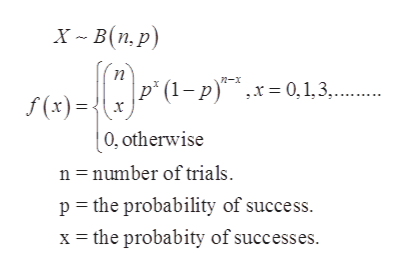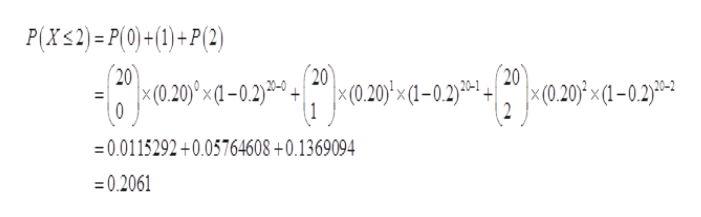# A university found that 20 percent of its students withdraw without completing the introductory statistics course. Assume that 20 students registered for the courseA.  Do these 20 students constitute a binomial experiment? If yes, please justify by differentiating between success and failure B.  ompute the probability that 2 or fewer students will withdrawC.  Compute the probability that no more than 3 students will withdraw  *Please solve using the probability of successes and failures.  For example, NotationN=number of trialsP=Probability of successQ=Probability of failure*My professor told us not to use the Binomial Distribution Formula but instead to use  what's called a Binomial table**Please do not solve using Microsoft Excel.  My professor is not teaching using excel.  I would like to see the steps taken to getting the solution for my own reference to study.*

Question
21 views

A university found that 20 percent of its students withdraw without completing the introductory statistics course. Assume that 20 students registered for the course

A.  Do these 20 students constitute a binomial experiment? If yes, please justify by differentiating between success and failure

B.  ompute the probability that 2 or fewer students will withdraw

C.  Compute the probability that no more than 3 students will withdraw

*Please solve using the probability of successes and failures.  For example, Notation

N=number of trials

P=Probability of success

Q=Probability of failure

*My professor told us not to use the Binomial Distribution Formula but instead to use  what's called a Binomial table*

*Please do not solve using Microsoft Excel.  My professor is not teaching using excel.  I would like to see the steps taken to getting the solution for my own reference to study.*

check_circle

Step 1

Consider,

Sample size (n) = 20.

Proportion (p) = 0.20.

Step 2

A)

If X is a binomial random variable, then binomial probability distribution states that x is the number of success on a n number of trials of an experiment where each trial have the same probability and all trials are independent to each other.

The success in this case is number of students withdraw without completing the introductory statistics and the failure in this case is the number of students who do not withdraw completing the course.

The pdf of binomial distribution is as given below:help_outlineImage Transcriptioncloseх - В (п.р) п p (1-p)x 0,1,3,...... f(x) х 0, otherwise n number of trials p the probability of success. x the probabity of successes. fullscreen
Step 3

b)

The probability that two or ...help_outlineImage TranscriptioncloseP(X<2)= P(0)+(1)+P(2) 20 20 20 x(0.20) x(1-0.2)30- x(0,20)°xa-0.2)-+(0.20x1-0.2) + | x (0.20)x(1-02) 0 1 2 0.0115292+0.05764608 +0.1369094 =0.2061 fullscreen

### Want to see the full answer?

See Solution

#### Want to see this answer and more?

Solutions are written by subject experts who are available 24/7. Questions are typically answered within 1 hour.*

See Solution
*Response times may vary by subject and question.
Tagged in

### Other